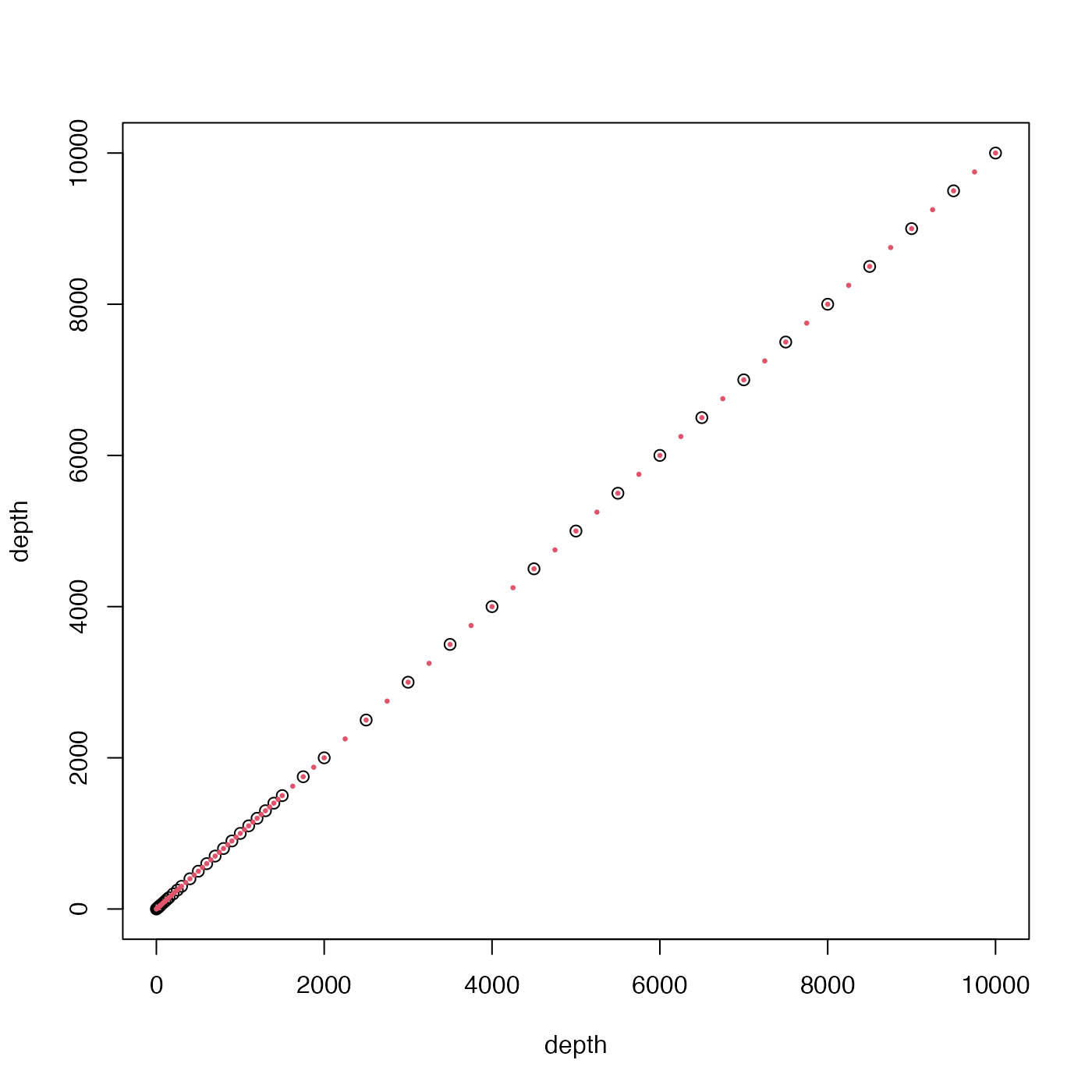This returns a vector of numbers that build upon the shorter lists provided in Chapter 10 of reference 1 and the more modern World Ocean Atlases (e.g. reference 2). With the default call, i.e. with n=0, the result is c(0, 10, 20, 30, 40, 50, 75, 100, 125, 150, 200, 250, seq(300, 1500, by=100), 1750, seq(2000, 10000, by=500)). For higher values of n, progressively more and more values are added between each pair in this sequence. See the documentation for sectionGrid() for how standardDepths can be used in gridding data for section plots.

standardDepths(n = 0)

## Arguments

n

Integer specifying the number of subdivisions to insert between each of the stated levels. For exmple, setting n=1 puts a 5m level between the 0 and 10m levels, and n=2 puts 3.33 and 6.66 between 0 and 10m.

## Value

A vector of depths that are more closely spaced for small values, i.e. a finer grid near the ocean surface.

Dan Kelley

## Examples

depth <- standardDepths()
depth1 <- standardDepths(1)
plot(depth, depth)
points(depth1, depth1, col = 2, pch = 20, cex = 1 / 2)